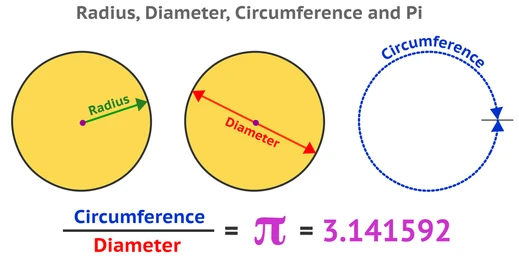Ratio of circumference and diameter of circle

## Objective

To find the ratio of circumference and diameter of a circle.

## Theoryi.e. C/d = π

Ratio of circumference and diameter of circle.

The property of a circle states that the ratio of circumference and diameter of a circle is always nearly equals to pii.

## Pre-requisite Knowledge

### Circle

In Geometry, A circle is the locus of all points in a plane which are equidistant from a fixed point. A circle is a collection of points that are at a fixed distance from the center of the circle. A circle is a closed geometric shape. We see circles in everyday life such as a wheel, pizzas, a circular ground, etc. The measure of the space or region enclosed inside the circle is known as the area of the circle.

Diameter is double of the radius of circle i.e. r=2d

Circumference of circle is equals 2πr.

Area of circle is π*r*r.